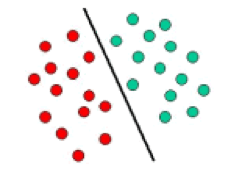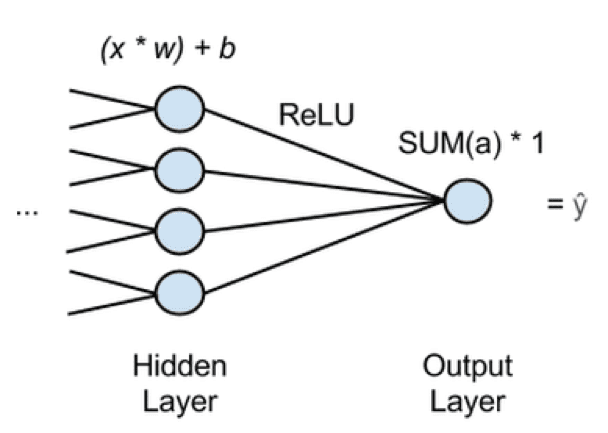Topics: AI | Data Science | Data Visualization | Deep Learning | Machine Learning | NLP | Python | R | Statistics

KDnuggets Home » News » 2017 » Jun » Tutorials, Overviews » The Machine Learning Algorithms Used in Self-Driving Cars ( 17:n24 )

#The Machine Learning Algorithms Used in Self-Driving Cars

http likes 379

Machine Learning applications include evaluation of driver condition or driving scenario classification through data fusion from different external and internal sensors. We examine different algorithms used for self-driving cars.

By Savaram Ravindra, Tekslate.com.

Today, the machine learning algorithms are extensively used to find the solutions to various challenges arising in manufacturing self-driving cars. With the incorporation of sensor data processing in an ECU (Electronic Control Unit) in a car, it is essential to enhance the utilization of machine learning to accomplish new tasks. The potential applications include evaluation of driver condition or driving scenario classification through data fusion from different external and internal sensors – like lidar, radars, cameras or the IoT (Internet of Things).

The applications that run the infotainment system of a car can receive the information from sensor data fusion systems and for example, have the capability to direct the car to a hospital if it notices that something is not right with the driver. This application based on machine learning also includes the driver’s speech and gesture recognition and language translation. The algorithms are classified as an unsupervised and supervised algorithms. The difference between both of them is how they learn.

The Supervised algorithms make use of a training dataset to learn and they continue to learn till they get to the level of confidence they aspire for (the minimization of the probability of error). The supervised algorithms can be sub-categorized into regression, classification and anomaly detection or dimension reduction.

The Unsupervised algorithms try to derive value from the available data. This implies, within the available data, an algorithm develops a relation in order to detect the patterns or divides the data set into subgroups depending on the level of similarity between them. The Unsupervised algorithms can be largely sub-categorized into association rule learning and clustering.

The reinforcement algorithms are another set of machine learning algorithms which fall between unsupervised and supervised learning. For each training example, there is a target label in supervised learning; there are no labels at all in unsupervised learning; the reinforcement learning consists of time-delayed and sparse labels – the future rewards.

The agent learns to behave in environment depending on these rewards. To understand the limitations and merits of an algorithm and to develop efficient learning algorithms is the goal in reinforcement learning. The reinforcement learning potentially addresses a huge number of practical applications that range from problems in AI to the control engineering or operations research – all that are relevant for the development of a self-driving car. This can be categorized as indirect learning and direct learning.

In the autonomous car, one of the major tasks of a machine learning algorithm is continuous rendering of surrounding environment and forecasting the changes that are possible to these surroundings. These tasks are classified into 4 sub-tasks:

• The detection of an Object
• The Identification of an Object or recognition object classification
• The Object Localization and Prediction of Movement

The machine learning algorithms are loosely divided into 4 classes: decision matrix algorithms, cluster algorithms, pattern recognition algorithms and regression algorithms. One category of the machine learning algorithms can be utilized to accomplish 2 or more subtasks. For instance, the regression algorithms can be utilized for object localization as well as object detection or prediction of the movement.Decision Matrix Algorithms

The decision matrix algorithm systematically analyzes, identifies and rates the performance of relationships between the sets of information and values. These algorithms are majorly utilized for decision making. Whether a car needs to brake or take a left turn is based on the level of confidence these algorithms have on recognition, classification and prediction of the next movement of objects. The decision matrix algorithms are models composed of various decision models trained independently and in some way, these predictions are combined to make the overall prediction, while decreasing the possibility of errors in decision making. AdaBoosting is the most commonly used algorithm.

Adaptive Boosting or AdaBoost is a combination of multiple learning algorithms that can be utilized for regression or classification. It overcomes overfitting when compared with any other machine learning algorithms and is often sensitive to outliers and noisy data. In order to create one composite powerful learner, AdaBoost uses multiple iterations. So, it is termed as adaptive. By adding the weak learners iteratively, AdaBoost creates a strong learner. A new weak learner is appended to the entity and a weighing vector is adjusted in order to pay attention on examples that were classified incorrectly in the prior rounds. A classifier that has much higher accuracy than the classifiers of weak learners is the result.AdaBoost helps in boosting the weak threshold classifier to strong classifier. The above image depicts the implementation of AdaBoost in one single file with understandable code. The function contains a weak classifier and the boosting component. The weak classifier attempts to locate the ideal threshold in one of data dimensions to segregate the data into 2 classes. The classifier is called by the boosting part iteratively and after each classification step, it changes the weights of misclassified examples. Because of this, a cascade of weak classifiers is created and it behaves like a strong classifier.

Clustering Algorithms

Sometimes, the images acquired by the system are not clear and it becomes difficult to locate and detect objects. Sometimes, there is a possibility of classification algorithms missing the object and in that case, they fail to categorize and report it to the system. The possible reason could be discontinuous data, very few data points or low-resolution images. The clustering algorithm is specialized in discovering the structure from data points. It describes the class of methods and class of problem like regression. The clustering methods are organized typically by modeling the approaches like hierarchical and centroid-based. All methods are concerned with utilizing the inherent structures in data to organize the data perfectly into groups of maximal commonality. K-means, Multi-class Neural Network is the most commonly used algorithm.

K-means

K-means is a famous clustering algorithm. K-means stores k centroids that it utilizes for defining the clusters. A point is said to be in a specific cluster if it is closer to the centroid of that cluster than any other centroid. By alternating between choosing the centroids depending on the current assignment of data points to clusters and assigning the data points to clusters depending on current centroids.K-means Algorithm – The cluster centroids are depicted as crosses and training examples are depicted as dots. (a) Original dataset. (b) Random initial cluster centroids. (c-f) The demonstration of running 2 iterations of k-means. Each training example is assigned in each iteration to the cluster centroid that is closest and then, each cluster centroid is moved to mean of points assigned to it.

Pattern Recognition Algorithms (Classification)

The images obtained through sensors in Advanced Driver Assistance Systems (ADAS) consists of all kinds of environmental data; filtering of the images is needed to determine the instances of an object category by ruling out the data points that are irrelevant. Before classifying the objects, the recognition of patterns is an important step in a dataset. This kind of algorithms are defined as data reduction algorithms.

The data reduction algorithms are helpful in reducing the dataset edges and polylines (fitting line segments) of an object as well as circular arcs to edges. Till a corner, the line segments are aligned with the edges and a new line segment will begin after this. The circular arcs align with the line segments’ sequences that is similar to an arc. In various ways, the features of the image (circular arcs and line segments) are combined to form the features that are utilized for determining an object.

With the PCA (Principle Component Analysis) and HOG (Histograms of Oriented Gradients), the SVM (Support Vector Machines) are the commonly used recognition algorithms in ADAS. The K nearest neighbor (KNN) and Bayes decision rule are also used.

Support Vector Machines(SVM)

SVM are dependent on the decision planes concept that define the decision boundaries. The decision plane separates the object set consisting of distinct class memberships. A schematic example is illustrated below. In this, the objects belong to either RED or GREEN class. A boundary line of separation separates the RED and GREEN objects. Any new object that falls to the left is labeled as RED and it is labeled as GREEN if it falls to the left.Regression Algorithms

This kind of algorithm is good at predicting events. The Regression Analysis evaluates the relation between 2 or more variables and collate the effects of variables on distinct scales and are driven mostly by 3 metrics:

• The shape of regression line.
• The type of dependent variables.
• The number of independent variables.

The images (camera or radar) play a significant role in ADAS in actuation and localization, while for any algorithm, the biggest challenge is to develop an image-based model for feature selection and prediction.

The repeatability of the environment is leveraged by regression algorithms to create a statistical model of relation between the given object’s position in an image and that image. The statistical model, by allowing the image sampling, provides fast online detection and can be learned offline. It can be extended furthermore to other objects without the requirement of extensive human modeling. An object’s position is returned by an algorithm as the online stage’s output and a trust on the object’s presence.

The regression algorithms can also be utilized for short prediction, long learning. This kind of regression algorithms that can be utilized for self-driving cars are decision forest regression, neural network regression and Bayesian regression, among others.

Neural Network Regression

The neural networks are utilized for regression, classification or unsupervised learning. They group the data that is not labeled, classify that data or forecast continuous values after supervised training. The neural networks normally use a form of logistic regression in the final layer of the net to change continuous data into variables like 1 or 0.In the above figure, ‘x’ is the input, features passed forward from the previous layer of the network. Into every node of last hidden layer, many x’s will be fed and every x will be multiplied by w, a corresponding weight. To a bias, the products’ sum is added and moved to an activation function. An activation function is a ReLU (rectified linear unit), used commonly as it does not saturate on the shallow gradients like the sigmoid activation functions do. ReLU provides an output, activation a for each hidden node and the activations are added going into output node which passes the sum of activations. This implies, a neural network that performs regression contains single output node and this node will multiply the sum of activations of previous layer by 1. The network’s estimate, ‘y hat’ will be the result. ‘Y hat’ is the dependent variable that all the x’s map to. You can use the neural network in this way to obtain the function relating x(number of independent variables) to y (a dependent variable) that you are trying to predict.

Original post. Reposted with permission.

Bio: Savaram Ravindra is a Content Contributor at Tekslate.com, and earlier was a Programmer Analyst at Cognizant Technology Solutions. He holds a Masters degree in Nanotechnology from VIT University. He can be contacted at savaramravindra4@gmail.com.

Related:

Top Stories Past 30 Days
Most Popular
Most SharedGet KDnuggets, a leading newsletter on AI, Data Science, and Machine Learning